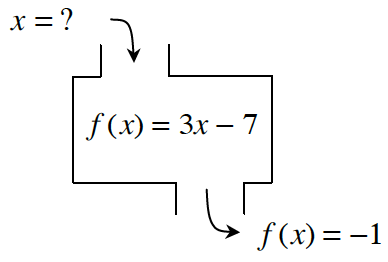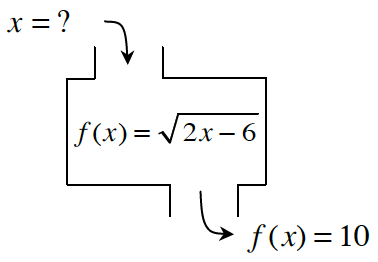Home > CCAA8 > Chapter cca1 > Lesson cca1.2.5 > Problem1-80

1-80.

Find the inputs for the following functions with the given outputs. If there is no possible input for the given output, explain why not. Homework Help ✎

1.Write out the equation.
$−1=3x−7$

Solve for $x$.
$6=3x$

$2=x$

1.Follow the steps in part (a).

$x=53$